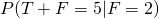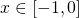# Post- 2015 H2 Mathematics Paper 1 ThoughtsEasier than 2014. Tricky. Very tricky. Tedious.

When I did it, it felt a bit like a AJC paper slightly, so I believe AJC students might find the paper quite comfortable. Paper is not super conceptual.

Highlights: Question 3 which test students on the definition of integration, not a difficult question, but some may struggle to express their answers. Piecewise function was expected, as I told my students. Truck load of integration, technique no good means you struggle already.

### So what should we expect in paper 2?

#### Differential Equation

Start you off with a differential equation then have you find the equation, perhaps draw a graph and comment about the long run? Remember to state if k is positive or negative constant.
How it can be hard, getting you to find the equation and link it with conics. Try finding the equation of curve whose normal to the curve passes through.

Probably give it a 7-10 marks.

#### Vectors

Clearly about planes, projection and perpendicular distance. Students should familiarise themselves with the conditions for various situations for plane (intersecting at a common line or point). And if they want a set of values, be alert. Same for coordinates.
How it can be hard, when they start reflecting the lines and giving you an angle, and ask you to hence.

Probably give it a 10 marks at least.

#### Mathematical Induction

I doubt a trigonometry one will repeat itself. Paper 1 did test student enough trigonometry in the last question. What remains is either a conjecture, or recurrence MI. So please go revise how to key a sequence into the GC, how to show increasing/ decreasing sequence. For conjecture, students may find this post helpful. 🙂

How it can be hard, you can’t do your favourite MI if you can’t even do a conjecture.

Probably give it a 10 marks

#### Functions

I really hope they start putting some effort and put the fun in functions. Functions has been quite boring for the past years.

How it can be hard, put in some conics or ask you to find the range of composite, or restrict some domains such that the composite exists.

#### Complex Numbers

We know it will definitely still come out, and its gonna be Loci already. Be careful and if possible, learn how to check if your bisector or half-line should cut the origin or the centre of a circle, etc. Er don’t forget compass and protractor please.
How it can be hard, finding cartesian equations. Or simply giving you theand then ask you for exact coordinates.

Probably just 8 marks.

### Conclusion

Okay I know they don’t add up to 40 marks, but I’m not god, else I can tell you what the actual population mean mark will be. Give and take.

The paper should be tricky, so be careful with the unit, like(thousands) of people, ya.

As for statistics, definitions like the p-value, unbiased estimate, level of significance, necessary conditions for approximations and assumptions should be well memorised. Sampling, last time already la.

Students should know how to find things likegiven the distribution of T and F. Find the range of level of significance is an easy task but eludes some students still, partly because they overlook that we only rejectwhen, yes it is less than equal, not just less than. And we do not reject when, no more equal; I wrote this intuitive line cos just in case some blindly write equal again. Please take note, definitions are important in Mathematics.

For regression, I hope students know when to useto calculate sample values, and the intuition behind it. Also understand that r-value indicates correlation, and not causality, know that its independent of scaling and translation. You can read a bit here.

For a normal distribution, they should know that its centred aboutALWAYS. So learn to abuse the fact. Try a question here.

For PnC, just pray 🙂

Be precise and leave all answers like the regression line in 3SF, but use the 5SF to estimate values, etc.

And please be careful when you round off something in an inequality. :/ A good example will be the APGP question in 2015 H2 Math.

Now I need to get back to my lessons. But I do hope these help.

•aaa

hmm u say harder than 2013 but easier than 2014?? how can?

•AAAA

Just wondering if you have any idea what the past year’s A mark would roughly be? and your thoughts on the A mark for this paper 1?

•KS Teng

hi, I can’t really recommend a sufficient mark. :/ A necessary mark will be 70, while the SUPER safe mark is 85. I’ll reckon an 78 might be good. 🙂 And I know my student who got 81 (based on her calculations) got an A while another one who got 78 (based on her calculations too) got a B. :/ Then again, they did estimation too haha. so I’m not really sure if any overestimate or was too generous with her own marks. So hope this helps 🙂 Good luck for P2!

•Hi thank you so much for the information. Wonder for P1 where is the most common question parts to lose method marks? Bc I think 80% of my answers are correct but I’m not sure bout the method.

•KS Teng

question 1(i), human error in substitution. or some try to “simplify” the equation and made it worst. and its 3dp, 3dp, 3sf for answers.
question 3, for the explanation, when you dont link up the total area of n rectangles with the actual area. i dont see how the graph will be hard to guess out, a straight line curve works too.
question 4, can be careless for the weak students too due the overload of variables. or some round off 0.03125 to 0.0313. Never check second order derivative.
question 5, forgot to translate the graph 1 unit in positive x direction foror scaling the domain of x forquestion 6, forgot to sub a back in when findingcoefficient or even previously during the expansion.
question 7, not good at ratio and put 9:11
question 8, rounding off error when you’re finding t in (ii), a lot of weak students will overlook this as the rounding off with an inequality is counter intuitive. Not giving a set. A mistake students will repeat tomorrow when doing hypothesis testing if they didn’t realise.
question 10, last part, careless substitution when resolving definite integral
question 11, careless in differentiatingtoand forgetting to do implicit. Manipulation ofto find the non-trigo exact coordinates.

Thats why I think this paper isn’t that hard. mostly normal questions out to rob you of 1 mark here and there due to oversight. :/

•Student X

Does the rounding off for solving inequalities apply for all questions? All the answer keys I have seen for various questions always round off to the nearest 3 s.f regardless of the direction of the inequality sign.

•Student X

I mean most questions, not all

•KS Teng

normally, its okay, but you just need to make sure you dont disobey the inequality.

•KS Teng

no, i think if you read some school answer keys, when they round off, for instance for level of significance, they do need to rationalise. Else, you might end up with the wrong rejection decision.

Not readable? Change text.